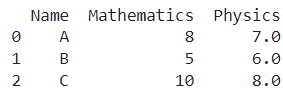# Pandas DataFrame: Replace Nan Values With Average Of Columns

A DataFrame is the primary data structure of the Pandas library in Python and is commonly used for storing and working with tabular data.

A common operation that could be performed on such data is to replace the NaN values in a Pandas DataFrame with the average of columns in order to update the existing information in it.

To start working with Pandas, we first need to import it:

``import pandas as pd``

We’ll also need the NumPy library for this to specify NaN values. To work with NumPy, we first need to import it in the following manner:

``import numpy as np``

## Running Example

Let us understand this operation with the help of an example. Consider the following DataFrame containing 3 students with names A, B, and C and their corresponding marks (out of 10) for two subjects, Mathematics and Physics.Code snippet for generating the above DataFrame :

``````import pandas as pd
import numpy as np

# Dictionary for our data
data = {'Name' : ['A', 'B', 'C'], 'Mathematics' : [8, 5, 10], 'Physics' : [np.nan, 6, 8]}

# DataFrame for the dictionary
df = pd.DataFrame.from_dict(data=data)

# Printing the DataFrame
print(df)``````

Here, data is a dictionary we created to initialize the DataFrame. For this, we use the DataFrame() function of the Pandas library which takes the dictionary as an argument and returns the required DataFrame. One important thing to note here is that the first row of the column physics has a NaN value.

Now, let’s say we need to replace the NaN values we have in the physics column with the average of values in that column in order to make the dataframe easier to handle.

The resulting output would look like this :Let us look at different ways of performing this operation on a given DataFrame :

## 1. Replace Nan Values of Pandas Column Using the fillna() function

In this method, we use the fillna() function to replace the nan values in a column of interest here physics with the average of values in that column.

First, we use df[‘Physics’] to access the physics column then we apply the fillna() function to it, and then use df[‘Physics’].mean() function to pass the average of the values in that column as a parameter to the fillna() function.

By default, the changes are not made in an in-place manner therefore reassignment is necessary. Let us take a look at the corresponding code snippet and generated output for this method:

``````import pandas as pd
import numpy as np

# Dictionary for our data
data = {'Name' : ['A', 'B', 'C'], 'Mathematics' : [8, 5, 10], 'Physics' : [np.nan, 6, 8]}

# DataFrame for the dictionary
df = pd.DataFrame.from_dict(data=data)

# Performing the operation
df['Physics'] = df['Physics'].fillna(df['Physics'].mean())

# Printing the DataFrame
print(df)``````

Output :Instead of using df[‘Physics’] to access the physics column, we can also use df.Physics to access it and still get the same results.

Let us take a look at the corresponding code snippet and generated output for this method:

``````import pandas as pd
import numpy as np

# Dictionary for our data
data = {'Name' : ['A', 'B', 'C'], 'Mathematics' : [8, 5, 10], 'Physics' : [np.nan, 6, 8]}

# DataFrame for the dictionary
df = pd.DataFrame.from_dict(data=data)

# Performing the operation
df.Physics = df.Physics.fillna(df.Physics.mean())

# Printing the DataFrame
print(df)``````

Output :In case we do not wish to perform reassignment and want to perform the operation in an in-place manner, we need to specify the parameter in place as True in the fillna() function.

Let us take a look at the corresponding code snippet and generated output for this method:

``````import pandas as pd
import numpy as np

# Dictionary for our data
data = {'Name' : ['A', 'B', 'C'], 'Mathematics' : [8, 5, 10], 'Physics' : [np.nan, 6, 8]}

# DataFrame for the dictionary
df = pd.DataFrame.from_dict(data=data)

# Performing the operation
df.Physics.fillna(df.Physics.mean(), inplace = True)

# Printing the DataFrame
print(df)``````

Output :## Conclusion

In this topic, we have learned to replace the nan values in a column of an existing Pandas DataFrame with the mean or average of values of that column, following a running example of test scores of students in different subjects, thus giving us an intuition of how this concept could be applied in real-world situations. Feel free to reach out to info.javaexercise@gmail.com in case of any suggestions.

Trending